# Linear Regression

Linear Regression is one of the most popular and frequently used algorithms in Machine Learning. According to a Kaggle survey, more than 80% of people from the machine learning community prefer this algorithm over others. So we might have got an idea about its popularity. Hence, to become an expert in the Machine Learning and data science domain, Linear Regression is essential. In this article, we will get a detailed overview of this algorithm.

## Key takeaways from this blog

After going through this blog, we will be able to understand the following things,

1. What is Linear regression in Machine Learning?
2. Mathematical understanding of the Linear Regression.
3. What are the types of Linear regression?
4. The loss function for Lineare Regression.
5. What is the Ordinary Least Squares (OLS) method?
6. How to measure the goodness of fit for Linear Regression?
7. What is polynomial regression, and why is it considered linear regression?
8. How to prepare a dataset to fit the Linear Regression model best?
9. A python-based implementation of Linear regression.
10. Possible interview questions on linear regression.

## What is Linear Regression in Machine Learning?

Linear Regression is a supervised machine learning algorithm that learns a linear relationship between one or more input features (X) and the single output variable (Y). As a standard paradigm of Machine Learning, we can say that the output variable is dependent on the input features.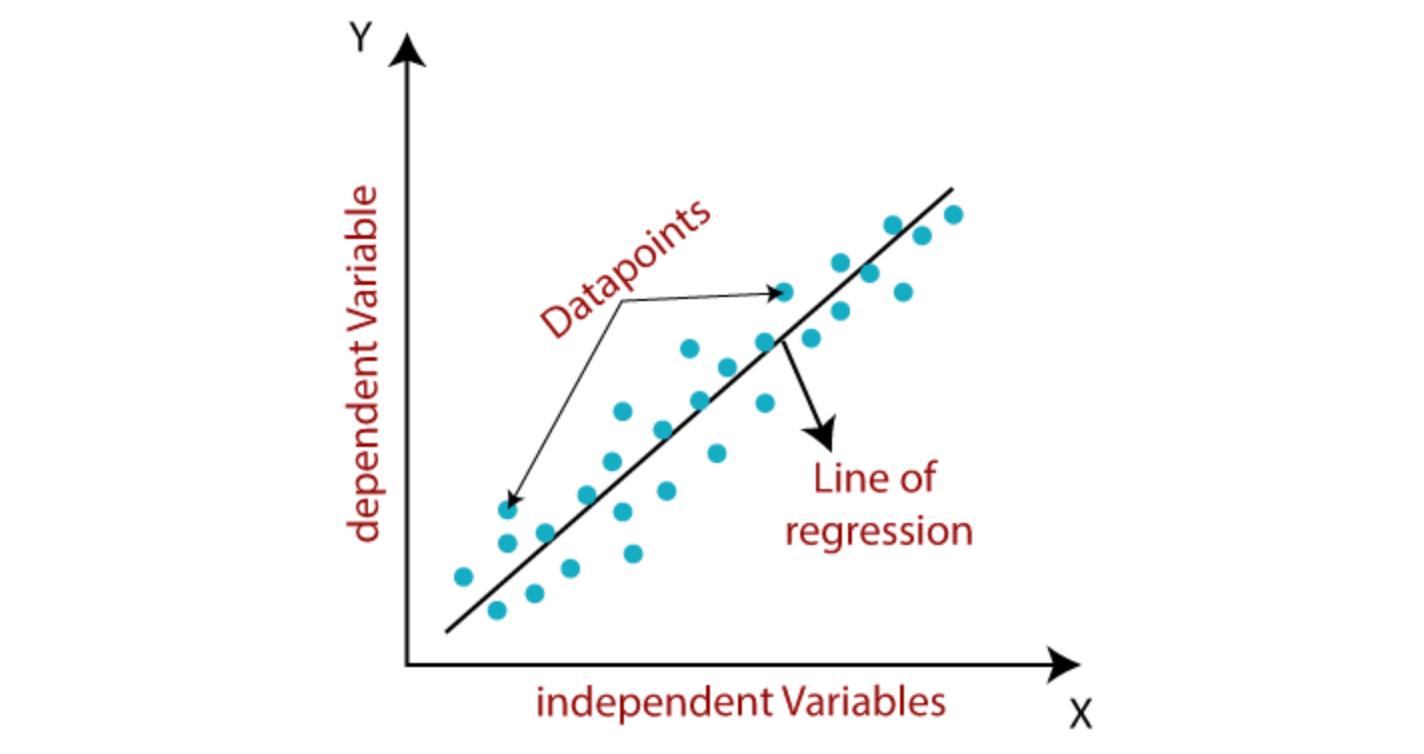## Mathematical Understanding of Linear Regression

In a machine learning problem, linearity is defined as the linearly dependent nature of a set of independent features X and the dependent quantity y. Mathematically, if X = [x1, x2, …, xn] is a set of independent features, and y is a dependent quantity, we try to find a function that maps y → X as,The βi's are the parameters (also called weights) that our Linear Regression algorithm learns while mapping X to Y using supervised historical data. ξ is the error due to fitting imperfection as we can not assume that all the data samples will perfectly follow the expected function.

### Example

Let's understand these mathematical terms via an example. Suppose we want to predict the price of any house. The crucial features that can affect this price are the size of the house, distance from the railway station/airport, availability of schools, etc. Let's treat all these records as a separate feature, then X1 = Size of house, X2 = Distance from the airport, X3 = Distance from school, and so on.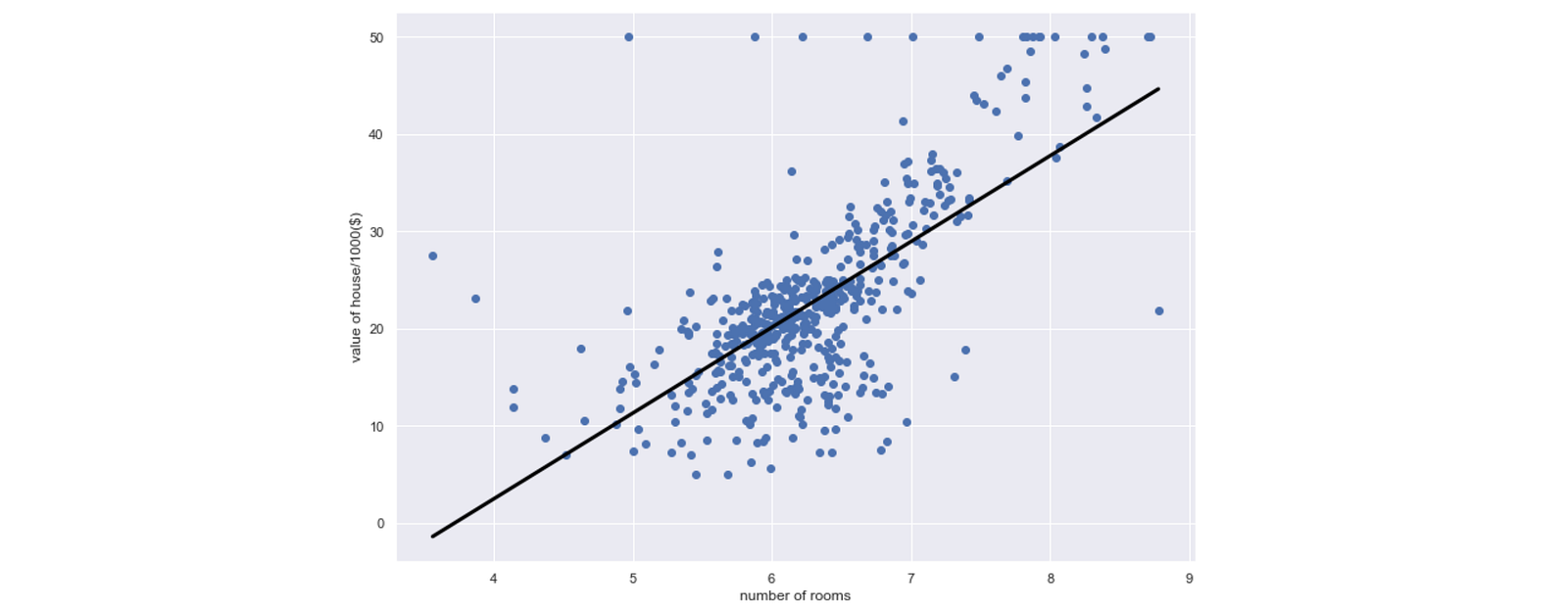Do all these features contribute equally to determining the house price? The answer would be No. Every feature has a certain weightage, like "size" matters the most and "distance from the airport" matters the least. Hence we need to multiply them with a real number describing their weightage, and βs in the above equation represent the same. Also, even if we learn the price prediction strategy, there will be minute differences in the predicted and actual prices of the house. The term ξ shows this imperfection in the above equation.

## What are the types of Linear Regression?

A model is linear when it is linear in parameters relating the input to the output variables. The dependency need not be linear in terms of inputs for the model to be linear. For example, all equations below are linear regression, and they define the model that represents the linear relationship between the model parameters.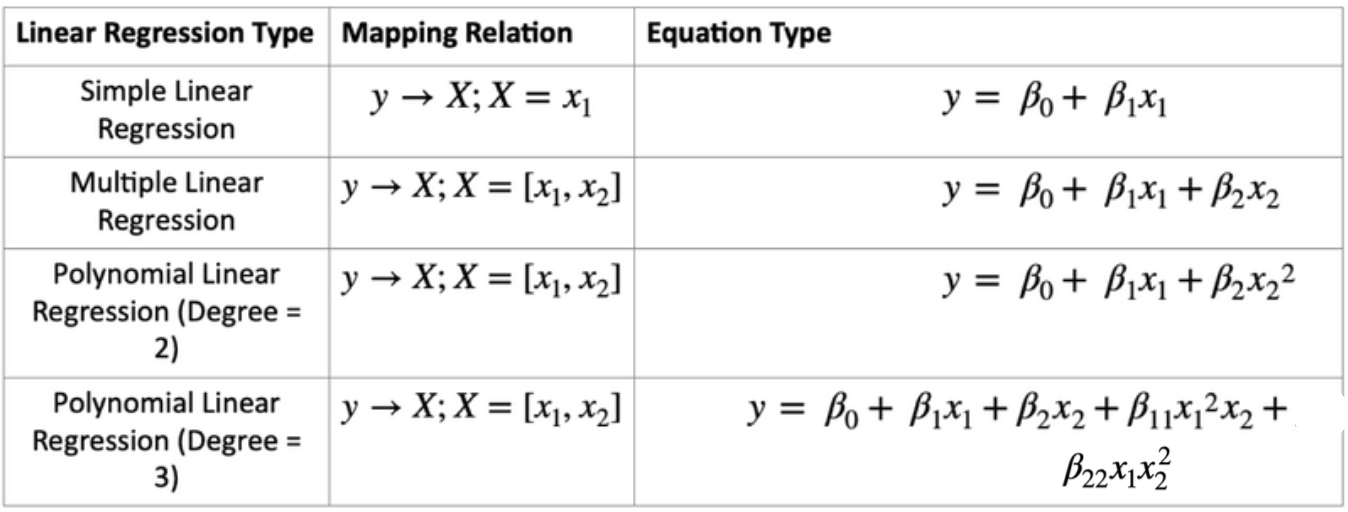### Simple Linear Regression

Suppose the number of independent features in X is just one. In that case, it becomes a category of simple linear regression where we are trying to fit a conventional linear line Y = m*X + c, where "m" is the slope and "c" is the intercept. For example, suppose we want to predict the house price by just knowing the size of the house.

### Multiple Linear Regression

If the number of independent features in X is more than 1, it becomes a category of multiple linear regression.For example, considering all essential features for predicting house price becomes a multiple linear regression. Most of the industry problems are based on this.

## Loss Function in Linear Regression

As we said, there will be some imperfections in the fitting. In the image below, the third sample, represented as a blue ball, shows the imperfection as ei.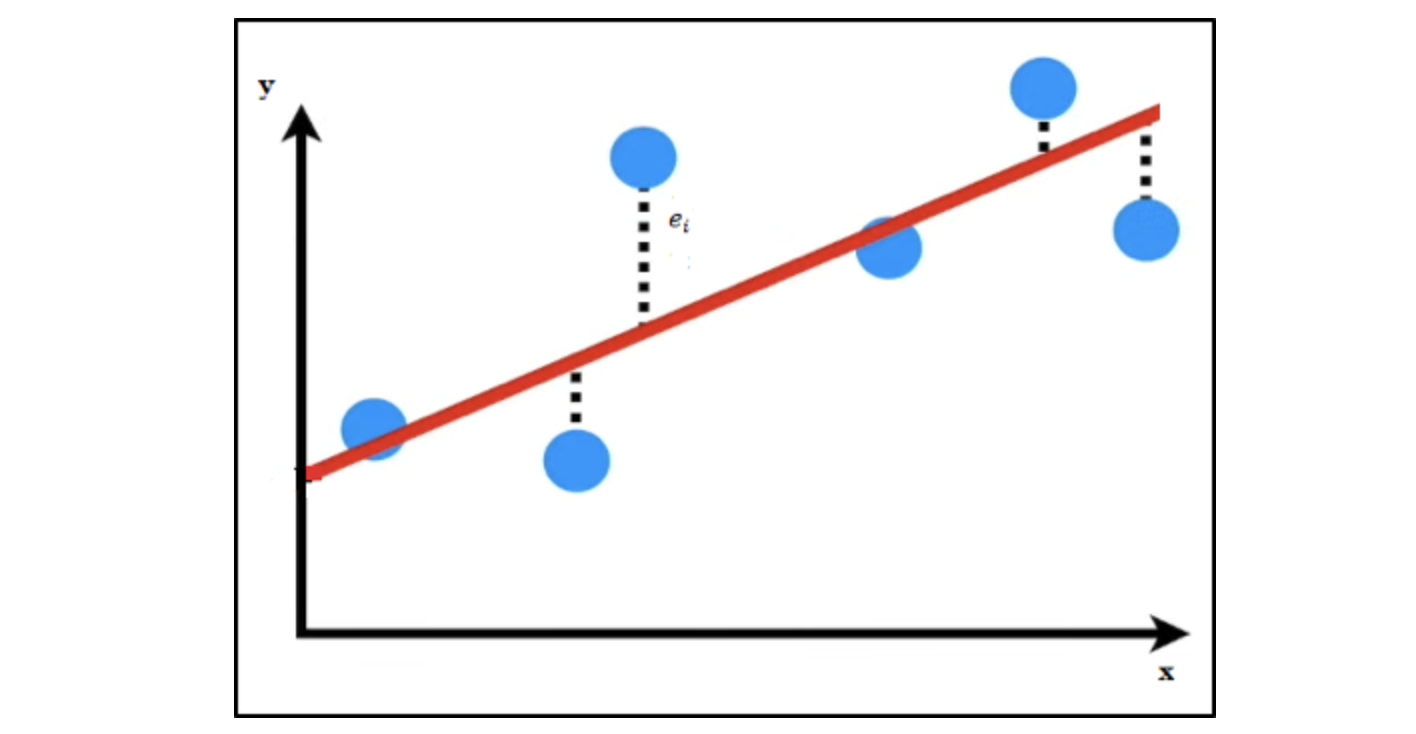The third sample has coordinates (Y, X1), and our linear regression model, which has learned the red line, predicts Y' for the same X1. Then the error (also known as residual) can be calculated as,This is for one sample, so if we go ahead and calculate the cumulative error for all the samples present in our dataset, it will be called the Loss function for our model. In a regression problem, the Sum of Squared Residuals (SSR) is one of the most common loss functions, where we sum up the squares of all the errors.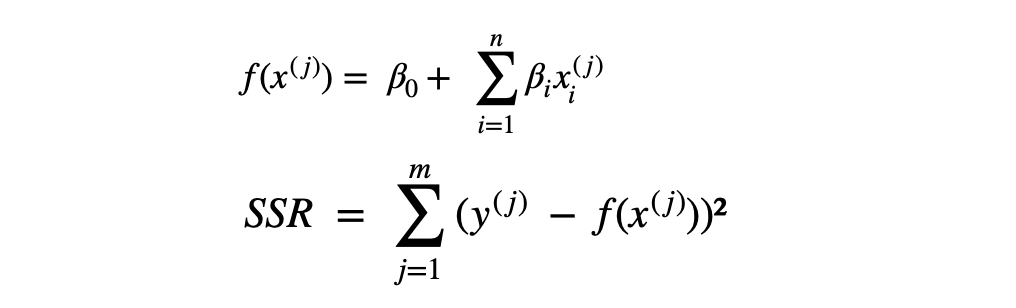### What is the Ordinary Least Squares (OLS) method?

When we fit the Linear regression model with this loss function, it varies the parameters (β0, β1, .., β1) and tries to find the best set of these parameters for which the average of the loss function becomes minimum. The loss function averaged over all the samples is called a Cost function for linear regression. With SSR as our loss function, finding the best set of parameters is termed the Ordinary Least Squares (OLS) method.

Finding the best parameters such that the cost would be minimum is an optimization problem and can be solved using several techniques like Gradient Descent, Linear Algebra, and differential calculus. To know the Gradient Descent method's working to solve this optimization problem, please look at this article.

## How to measure the goodness of fit for Linear Regression?

We are trying to solve a regression problem using the Linear Regression model, so several evaluation metrics, like MSE, RMSE, and R² score, can determine the goodness of fit. Formulas and their detailed explanation can be found here.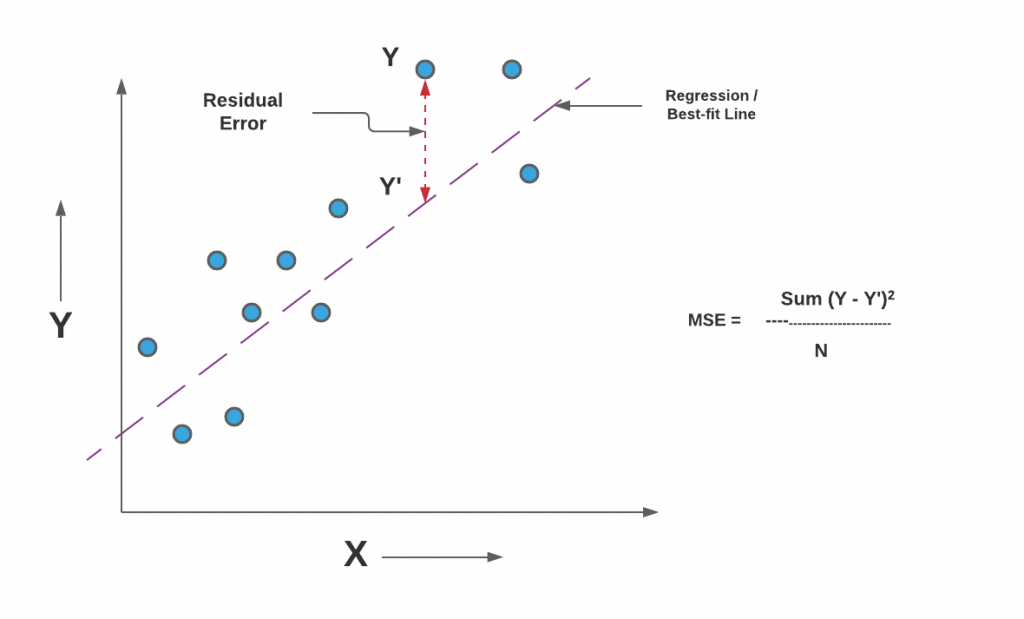## What is Polynomial Regression?

In real-life scenarios, there can be possibilities where a linear line won't fit the data perfectly. Independent variables do not possess a linear relationship with the dependant variable here, and hence we need our machines to learn a curvy relationship. When our machine learns this curvilinear trend, we call it a polynomial regression. The black line shows the linear fit on a non-linear trend in the image below; hence the performance was worst.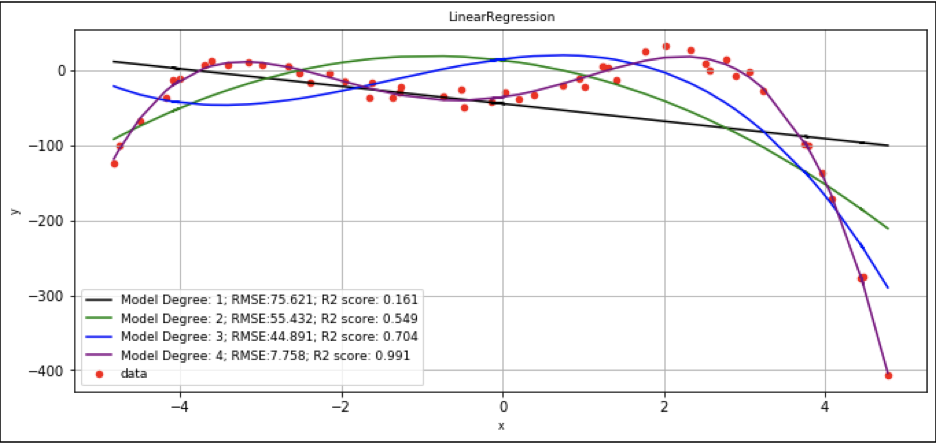## Why is polynomial regression a Linear Regression?

In polynomial regression, we try to fit a polynomial function with degrees >1, e.g., X², X³. So the mapping function would look like this,Please note that we can have multiple features (X1, X2 in the above equation), and function can contain higher degrees corresponding to any of the features. Let's take the case of multiple linear regression where we had multiple features present in our dataset and treat higher-order terms from the above equation as a new feature, let's say X3. Then the same equation will look like,This is precisely the same as the multiple linear regression case, so we consider polynomial regression as linear regression only.

## How to prepare a dataset to fit the Linear Regression model best?

As this algorithm has existed for more than 200 years, much research and studies have been done on this algorithm. The literature suggests different heuristics that can be kept in mind while preparing the dataset. As we discussed, Ordinary Least Square is the most common technique for implementing the Linear regression models, so one could try the methods below and see if the R² score is improving or not.

• Gaussian distributed data is more reliable: One can use different transformation techniques to fit the input data into a gaussian distribution as the prediction made by LR in such a case is more reliable.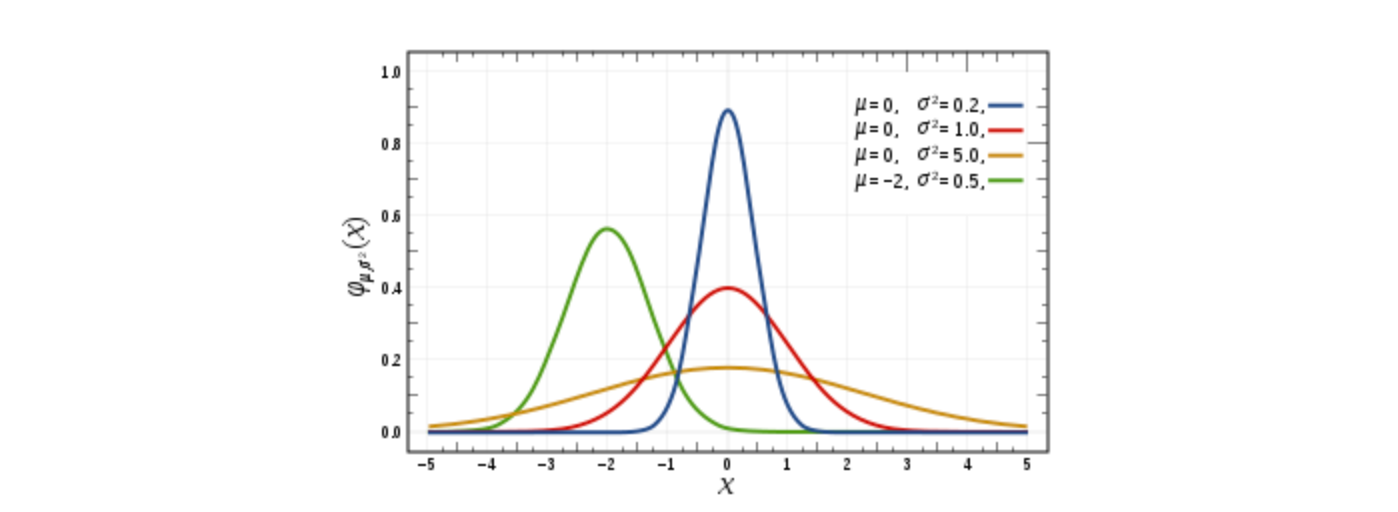• Outliers should be removed: In OLS (ordinary least square), we sum the residuals of all the data samples. In such a case, when there will be outliers present, the predictions will be biased, and eventually model will perform poorly.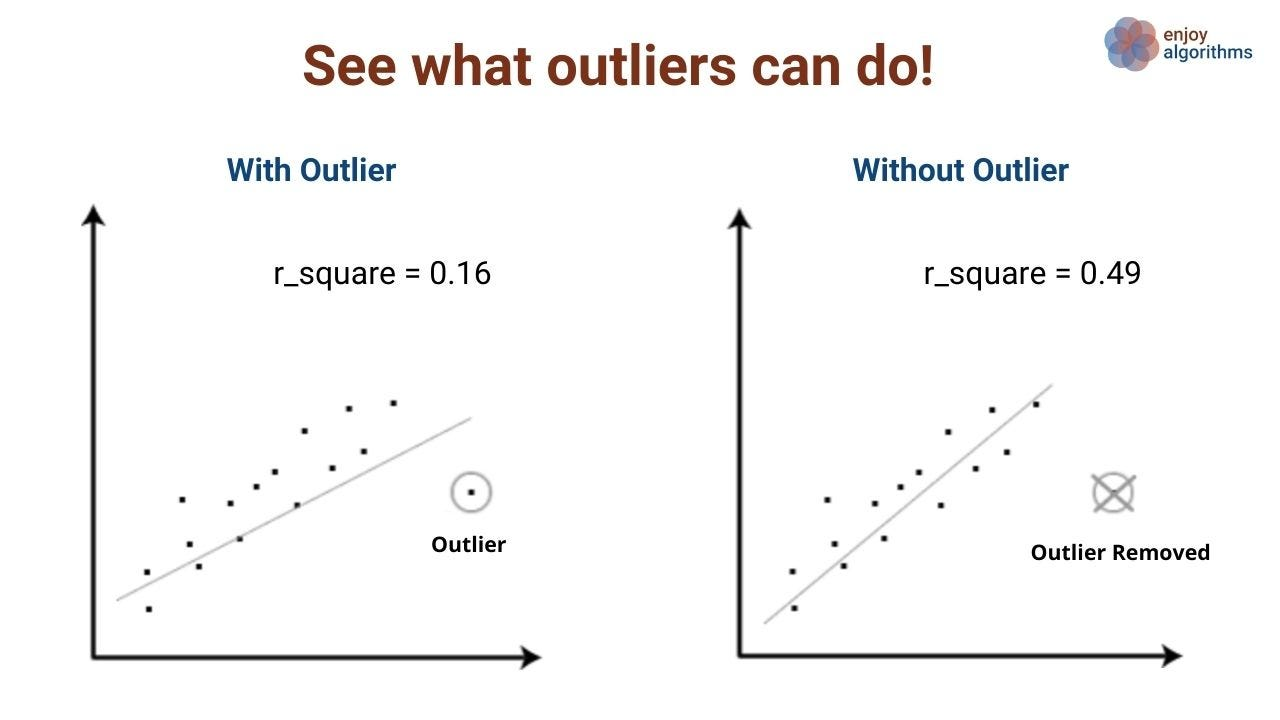• Input should be rescaled: Providing rescaled inputs either via standardization or normalization can produce more accurate results. This is more useful when there is a multiple-linear regression problem statement.
• Transformation of data for linearity between input and output: It should be ensured that the input and output are linearly related; otherwise, data transformation can make them linear, like logarithmic scaling.
• Colinearity should be removed: Model can be overfitted in case of colinearity. So with the help of correlation value, the most correlated can be removed for better generalization.

Too much theory now. Let's get some hands-on and implement the Linear Regression model.

## Python Implementation of Linear Regression

### Problem Statement

In our blog on coding machine learning from scratch, we discussed a problem statement on finding the relationship between input and output variables and wrote our program from scratch. But here, we will try to solve a similar but slightly complex problem of finding the below relationship from the recorded data samples of x and y using the Scikit-Learn library.### Step 1: Importing the necessary libraries

Required libraries to solve the problem statement are:

• numpy for algebraic operations
• matplotlib for plotting scattered data points and fitted curve
• LinearRegression from sklearn.linear_model for performing the regression operations
• Metrics such as r2_score to evaluate the goodness of fit of the performed regression
• Library for preprocessing features, such as PolynomialFeatures, to obtain higher input dimensions to process polynomial regression.
``````import numpy as np
import matplotlib.pyplot as plt
from sklearn.linear_model import LinearRegression
from sklearn.metrices import mean_squared_error, r2_score
from sklearn.preprocessing import PolynomialFeatures
``````

### Step 2: Data Formation

Machines learn from historical observations, but we can directly create data samples using a python program for this problem statement. A total of 50 data points are chosen from the same polynomial equation. We have added a randomized normally distributed noise value to avoid perfect fitting conditions to increase the complexity.The term np.random.normal(mean, variance, number of points) creates a normally distributed noise with the chosen mean and variance. We will add this noise in our original function to introduce the imperfection in data.

``````#creating and plotting dataset with curve-linear relationship
np.random.seed(0)

x = np.arange(-5,5,0.2) + 0.1*np.random.normal(0,1,50)

y = -1*(x**4) - 2*(x**3) + 13*(x**2) + 14*(x) - 24 + 10*np.random.normal(-1,1,50)

plt.figure(figsize=(10,5))
plt.scatter(x,y, color='red', s=25, label='data')
plt.xlabel('x',fontsize=16)
plt.ylabel('y',fontsize=16)
plt.grid()
plt.show()
``````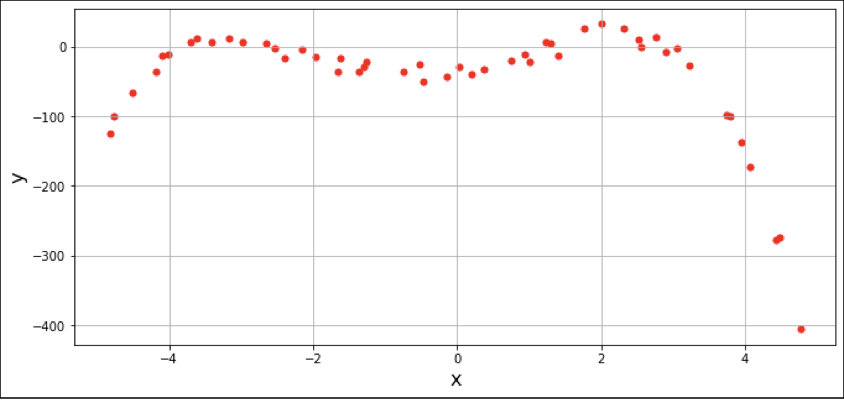### Step 3: Increasing the dimensionality of input variables

We have just the pair of X and Y values in our dataset. We need to increase the dimensionality in the dataset to perform the regression analysis. The function poly_data takes in input: the original data and the degree of the polynomial data and, based on the degree value, generates more features to perform the regression.

``````def poly_data(x,y,degree):
x = x[:,np.newaxis]
y = y[:,np.newaxis]

polynomial_features = PolynomialFeatures(degree=degree)
x_poly = polynomial_features.fit_transform(x)

return (x_poly,y)
``````

For example, poly_data(x,y,2) will generate a second order of the polynomial by mapping X → [1,x, x²], increasing the number of features for performing multiple regression.

### Step 4: Linear Regression Model Formation and Evaluation

PolyLinearRegression function takes the input data generated by the poly_data function, unpack it, and fits it in the LinearRegression model imported from Scikit-learn. Once the model is formed, we can use it for prediction.

The RMSE and R² scores are computed for the predicted output value. The formed model is a data structure similar to lists, hash, or queue, so it can easily store these evaluation metric values.

``````def PolyLinearRegression(data, degree=1):

x_poly,y = data

model = LinearRegression()
model.fit(x_poly, y) #it will fit the model

y_poly_pred = model.predict(x_poly) #prediction

rmse = np.sqrt(mean_squared_error(y,y_poly_pred))
r2 = r2_score(y,y_poly_pred)

model.degree = degree
model.RMSE = rmse
model.r2 = r2
return model
``````

### Step 5: Plotting the Results

Plotting the result for the regression analysis is done using the function Regression_plots, which returns the plot for a regression model of a different order.

``````def Regression_plots(data,model,axis):
x, y = data

axis.plot(x[:,1],model.predict(x), color=color[degree=1],
label = str("Model Degree: %d"%model.degree)
+ str("; RMSE:%.3f"%model.RMSE
+ str("; R2 Score: %.3f"%model.r2))

axis.legend()
``````

### Step 6: Fitting various degree polynomials on the same data

We can not guess whether degree 3 or 4 will fit this dataset better by looking at the dataset. So we need to check different degree fitments and the RMSE and R² scores to find the best fitment. This section compiles the functions and plots to perform regression analysis of varying order polynomial functions. Polynomial degrees are varied from 1 to 4, and corresponding RMSE and R² values are present in the image below.

``````if __name__ == "__main__":
np.random.seed(0)

x = np.arange(-5,5,0.2) + 0.1*np.random.normal(0,1,50)
y = -1*(x**4) - 2*(x**3) + 13*(x**2) + 14*(x) - 24 + 10*np.random.normal(-1,1,50)

_,axis = plt.subplots()
color = ['black','green','blue','purple']
axis.grid()
axis.set_xlabel('x')
axis.set_ylabel('y')
axis.scatter(x[:,np.newaxis], y[:,np.newaxis], color='red',
s=25, label='data')
axis.set_title('LinearRegression")

for degree in range(1,5):
data = poly_data(x,y,degree = degree)
model = PolyLinearRegression(data, degree=degree)
Regression_plots(data,model,axis)

``````

### Observation

It is observed that when we increase the model's degree, the RMSE is reducing, and the R² score is improving, which means the higher dimension polynomials fit the data better compared to the lower dimensions.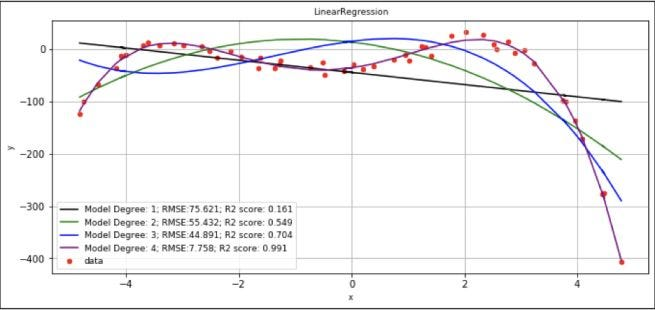## Hyperparameters for Linear regression

If we keep increasing the polynomial degree, we will suddenly land in the overfitting problem. In our case, we fabricated the data, and hence we know that the polynomial with degree 4 will fit it perfectly. But, in real scenarios, the fitting degree of the polynomial can not be guessed by looking at the scatter plot of data samples. Here, we keep on increasing the degree, and when the model overfits, we use regularization techniques to cure it. While applying the regularization, a hyper-parameter of lambda needs to be tuned.

## Industrial Applications of Linear Regression

Linear Regression is the most loved machine learning algorithm. Some most popular industrial applications of this algorithm are:

1. Life Expectancy Prediction: Based on geographical and economic factors, World Health Organisation tries to predict the average life of any living thing.
2. House price prediction: Based on the factors like size of the house, locality, and distance from the airport, the real-estate business tries to expect the price of any home.
3. Forecasting the sales for companies: Based on the previous months' sales, Linear Regression can predict the coming months' sales.

## Possible Interview Questions

Linear regression is the most used algorithm in machine learning and data science. In every interview for machine learning engineer or data scientist positions, interviewers love to ask questions to check the basic understanding of how this algorithm works. Some of those questions can be,

1. What is a Linear Regression algorithm, and Why do we say it is a Machine Learning algorithm?
2. What is the Ordinary Least Square method?
3. What are residuals?
4. If input variables are not linearly related, can it still be a linear regression algorithm?
5. How to ensure the better performance of Linear regression models?

## Conclusion

In this article, we discussed the most famous algorithm in machine learning, i.e., Linear regression. We implemented the linear regression model on our constructed data step-wise to understand all the verticals involved. We also discussed the methods using which we can increase the performance of the linear regression model. We hope you enjoyed it.